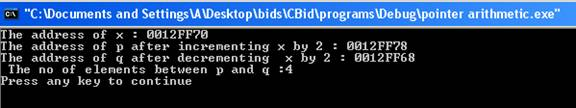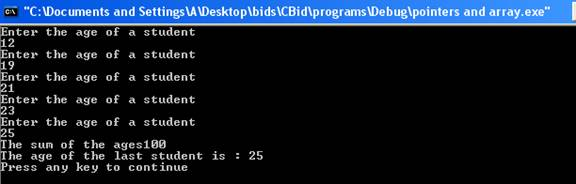Pointers

Before reading this tutorial, you should have knowledge of arrays.

A pointer is a variable that is used to store a memory address. The address is the location of the variable in the memory. Pointers help in allocating memory dynamically. Pointers improve execution time and saves space.  Pointer points to a particular data type. The general form of declaring pointer is:-

type *variable_name;

type is the base type of the pointer and variable_name is the name of the variable of the pointer. For example,

int *x;

x is the variable name and it is the pointer of type integer.

Pointer Operators

There are two important pointer operators such as ‘*’ and ‘&’. The ‘&’ is a unary operator. The unary operator returns the address of the memory where a variable is located.  For example,

int x*;

int c;

x=&c;

variable x is the pointer of the type integer and it points to location of the variable c. When the statement

x=&c;

is executed, ‘&’ operator returns the memory address of the variable c and as a result x will point to the memory location of variable c.

The ‘*’ operator is called the indirection operator. It returns the contents of the memory location pointed to.  The indirection operator is also called deference operator. For example,

int x*;

int c=100;

int p;

x=&c;

p=*x;

variable x is the pointer of integer type. It points to the address of the location of the variable c. The pointer x will contain the contents of the memory location of variable c. It will contain value 100. When statement

p=*x;

is executed, ‘*’ operator returns the content of the pointer x and variable p will contain value 100 as the pointer x contain value 100 at its memory location.  Here is a program which illustrates the working of pointers.

#include<iostream>

using namespace std;

int main ()

{

int *x;

int c=200;

int p;

x=&c;

p=*x;

cout << " The address of the memory location of x : " << x << endl;

cout << " The contents of the pointer x : " << *x << endl;

cout << " The contents of the variable p : " << p << endl;

return(0);

}

The result of the program is:-In the program variable x is the pointer of integer type. The statement

x=&c;

points variable x to the memory location of variable c. The statement

p=*x;

makes the contents of the variable p same as the contents of the variable c as x is pointing to the memory location of c.  The statement

cout << " The address of the memory location of x : " << x << endl;

prints the memory address of variable x which it is pointing to. It prints the hexadecimal address 0012FF78. This address will be different when the program is run on different computers. The statement

cout << " The contents of the pointer x : " << *x << endl;

prints the contents of memory location of the variable x which it is pointing to. The contents are same as the variable c which has value 200.  The statement

cout << " The contents of the variable p : " << p << endl;

has the same output 200 as the statement above. The contents of variable p is same as the contents of the pointer x.

Pointer Arithmetic

There are only two arithmetic operations that can be performed on pointers such as addition and subtraction. The integer value can be added or subtracted from the pointer. The result of addition and subtraction is an address. The difference of the two memory addresses results an integer and not the memory address. When a pointer is incremented it points to the memory location of the next element of its base type and when it is decremented it points to the memory location of the previous element of the same base type. For example,

int *x;

int *p;

p=x++;

here x and p are pointers of integer type. Pointer x is incremented by 1. Now variable p points to the memory location next to the memory location of the pointer x. Suppose memory address of x is 2000 and as a result p will contain memory address 2004 because integer type takes four bytes so the memory address is incremented by 4. Incrementing the pointer results in incrementing the memory address by the number of bytes occupied by the base type. For example,

double *x;

double *p;

p=x++;

variables x and p are pointers of double type. Pointer x is incremented by 1. Now if the memory address of x was 2000 and after incrementing p will contain memory address 2008 as double takes 8 bytes. Decrementing the pointer results in decrementing the memory address by the number of bytes occupied by the base type. You cannot add two pointers. No multiplication and division can be performed on pointers. Here is a program which illustrates the working of pointer arithmetic.

#include<iostream>

using namespace std;

int main ()

{

int *x;

int *p,*q;

int c=100,a;

x=&c;

p=x+2;

q=x-2;

a=p-q;

cout << "The address of x : " << x << endl;

cout << "The address of p after incrementing x by 2 : " << p << endl;

cout << "The address of q after derementing  x by 2 : " << q << endl;

cout << " The no of elements between p and q :" << a << endl;

return(0);

}

The result of the program is:-In the program x, p and q are pointers of integer type. The statement

p=x+2;

makes p to point to the memory address which is next two memory locations apart from the location of x.  The statement

q=x-2;

makes q to point to memory address which is previous two memory locations apart from the location of x. The statement

a=p-q;

computes the no of memory locations between p and q will come out to be 4. The statement

cout << "The address of x : " << x << endl;

prints the address of the memory location of x which is 0012FF70. The statement

cout << "The address of p after incrementing x by 2 : " << p << endl;

prints the memory address of p which comes out to be 0012FF78. Each memory location occupies 4 bytes. Therefore after incrementing by 2, memory address is incremented by 8. The statement

cout << "The address of q after decrementing  x by 2 : " << q << endl;

prints the memory address of q which is 0012FF68. After decrementing, the memory address is decremented by 8. The statement

cout << " The no of elements between p and q :" << a << endl;

prints the no of memory locations between p and q which comes out to be 4 as p points to next two memory locations of x and q points to the previous two memory locations of x.

Pointers and Arrays

The pointers and arrays have a very close relationship. The pointer can be set to the address of the first element of the array. For example,

int age[];

int *p;

p=&age;

p will point to the address of the first element of the array. For example

(p+4)

will point to the fifth element of the array.  Pointers are helpful where size of the array is unknown. Declaring the array with a size of large value wastes lot of space. Pointers improve the execution time. Here is a program which illustrates the working of arrays and pointers.

#include<iostream>

using namespace std;

int main()

{

int age;

int *p;

int sum=0,i;

char yes='y';

p=age;

for(i=0;i<5;i++)

{

cout << "Enter the age of a student" << endl;

cin >> *p;

sum=sum+*p;

p++;

}

p=age;

cout << "The sum of the ages" << sum << endl;\

cout << "The age of the last student is : " << *(p + 4) << endl;

return(0);

}

The result of the program is:-The array age is of integer type. The pointer p points to the first element of the array.

p=age;

The user is allowed to enter the age of the student. The statement

cin >> *p;

stores the age of the person in contents of the array.  The pointer is incremented by one memory location so that next time age is stored in new memory location.

p++;

sum of the ages is calculated. The pointer is again referred to the address of the first element of the array. The age of the last student cab be accessed using *(p+4) which contains the value of the 5th element of the array.

After an introduction of arrays, let us move on to discuss functions.

Go to the previous lesson or proceed to the next lesson